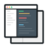# Anomaly Detection Using Deep Learning and Autoencoders

##### Deep Learning Project- Learn about implementation of a machine learning algorithm using autoencoders for anomaly detection.#### Videos

Each project comes with 2-5 hours of micro-videos explaining the solution.#### Code & Dataset## What will you learn

Understanding the problem statement
Importing the dataset and importing libraries
Performing basic EDA and checking for null values
Imputing the null values filling them using appropriate method
Using ggplot to visualize the Dataset
Importing h2o library and initializing an h2o cluster
Splitting Dataset into Train and Test
Defining parameters for training a Neural Network
Training the neural network for making predictions
Understanding what is difference between Artificial Neural Networks and Autoencoders
How does an Autoencoder work
How to Autoencode a pre-trained Neural Networks
Visualizing the effectiveness of an Autoencoded model and a Neural Networks using ggplot
Making predictions using the trained model

## Project Description

Deep learning is an upcoming field, where we are seeing a lot of implementations in day-to-day business operations, including segmentation, clustering, forecasting, prediction or recommendation etc. Deep learning architecture has many branches and one of them is the deep neural network (DNN), the method that we are going to analyze in this deep learning project is about the role of Autoencoders in performing classification and optimizing the hyperparameters.

## Similar Projects

#### Predict Census Income using Deep Learning Models

In this project, we are going to work on Deep Learning using H2O to predict Census income.

#### Solving Multiple Classification use cases Using H2O

In this project, we are going to talk about H2O and functionality in terms of building Machine Learning models.

## Curriculum For This Mini Project

Problem Statement
03m
01m
What is Deep Learning
05m
First Steps
01m
Univariate & Bivariate examples
04m
Correlation between variables
07m
Logistic Regression to predict Anomaly
05m
Compute ROC curve from Logistic Regression Model
05m
Train, test and validation of the model
03m
Deep Learning Models to predict Anomaly
02m
Rattle
10m
04m
Deep Learning Libraries in R
06m
MXNet Library
16m
H2O Library
14m
Data Exploratory Stage
10m
Initialize the Model
03m
Create Data Partition
04m
Apply AutoEncoders
07m
Analyse Model Result
08m